Regression
We can distinguish between t\\ 0 types of analysis in experiments involving two variables. say, x and y. In the first type, called correlation analysis, both variables are random, and we are interested in finding the relation between them. An
example is the analysis of the relation, if any. between the chemistry grades x and physics grades y of a group of students. We will not deal with correlation analysis in this text, because it is an advanced topic in statistics. With the second type of analysis, called regression. one of the variables. say, x, is regarded as an ordinary variable because we can measure it without error or assign it whatever values we want. The variable x is the independent
or controlled variable. The variable y is a random variable. Regression analysis deals with the relationship between)’ and .r , An example i:; the dependence of the outdoor temperature y on the time of day .r. In the previous section we used the MATLAB function poly fit to perform regression analysis with functions that ‘are linear or could be converted to linear form by a logarithmic or other transformation. The poly fit function is based on the least squares method. which we now discuss. We also show how to use this function to develop polynomial and other types of functions.

The Least Squares Method
Suppose we have the three data points given in the following table, and we need to determine the coefficients of the straight line y = IIlX + b that best fit the following data in the least squares sense.According to the least squares criterion, the line that gives the best fit is the one that minimizes J, the SUI11 of the squares of the vertical differences between the line and the data points (see Figure 5.6-1). These differences are called the residuals. Here there are three data points, and J is given byyou are familiar with calculus, you know that the values of m and b that minimize J are found by setting the partial derivatives aJ lam and aJ /ab equal to zero.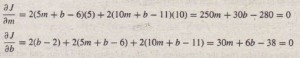These conditions give the following equations that must be solved for the two unknowns m and b.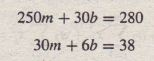The solution is III = 0.9 and b = 11/6. The best straight line in the least squares sense is y = 0.9x + 11/6. If we evaluate this equation at the data values x = 0,5, and 10, we obtain the values y = 1.833,6.333, 10.8333. These values are different from the given data values y = 2, 6, and 11 because the line is not a perfect fit to
the data. The value of J is J = (1.833 – 2)2 +(6.333 – 6)2 +(10.8333 – 11)2 = 0.16656689. No other straight line will givea lower value of J for this data. This line is shown in Figure 5.6-2.

Now suppose we want to fit a quadratic function y = alx2 + a2x+ a) to a set of m data points. Then the expression for J isUsing calculus (find the derivatives aJ jaa), aJ jao2.and aJ jaa3 and set them equal to 0). we can obtain the following equations that must be solved for al. a2. and a3:These three linear equations are in terms of the three unknowns al. a2.and a3. and they can be solved easily in MATLAB.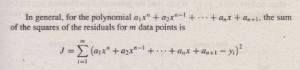The values of the 11 + I coefficients a; that minimize J can be found by solving a set of 11 + I linear equations. The polyf it function provides this solution. Its syntax is p = poly fit (x , y r n ) . The function fits a polynomial of degree 11 to data described by the vectors x and y, where x is the independent variable. The result p is the row vector of length 11 + I that contains the polynomial coefficients in order of descending powers. Table 5.6-1 summarizes the polyf it and polyval functions. To ilJustrate the use of the polyfi t function, consider the data set where x = I, 2, 3, … , 9 and y = 5,6, 10,20, 28, 33, 34, 36,42. The following script file finds and plots the first- through fourth-degree polynomials for this data and evaluates J for each polynomial.

x = [1:9);
y = [5,6(10,20,28.33,34,36,42);
xp = [1:0 .01 :9) ;
for k = 1:4
coeff = polyfit(x,y,k)
yp(k,:) = polyval(coeff,xp);
J(k) = surn((polyval(coeff,x)-y) .A2J;
end
subp Lot t 2,2, 1)
plot(~p,ypp,:J,x,y,’o’J,axis([O 10 0 SOH

subplot(2,2;2)
plot(xp,yp(2,:),x,y,’o’),axis([0 10 0 50))
subplot(2,2,3)
plot(xp,yp(3,:),x,y,’o’),axis([0 10 0 50))
subplot(2,2,4)
plot(xp,yp(4,:),x,y,’o’),axis([0 10 0 50))
disp(J)

The plots are shown in Figure 5.6-3. and the J values are, to two significant figures. 72. 57, 42, and 4.7. Thus the value of J decreases as the polynomial degree is increased, as we would expect. The figure shows why the fourth-degree polynomial can fit the data better than the lower-degree polynomials. Because it has more coefficients, the fourth-degree polynomial can follow the “bends” in the data more easily than the other polynomials can. The first-degree polynomial (a straight line) cannot bend at all, the quadratic polynomial has one bend, the cubic has two bends, and the quartic has three bends. The polynomial coefficients in the preceding script file are contained in the vector po ly fit (x , y r k ) . If you need the polynomial coefficients, say, for the cubic polynomial, type poly fit (x , y, 3) after the program has been run.An example of a fifth-degree polynomial that passes through all six
data points but exhibits large excursions between points.

The result is -0.1019,1.3081,0.7433, and 1.5556. These values correspond to the polynomial -0.1019×3 + 1.308Ix2 +0.7433x + 1.5556. It is tempting to use a high-degree polynomial to obtain the best possible fit. However, there are two dangers in using high-degree polynomials. Figure 5.6-4 illustrates one of these dangers. The dtta values are x ::±: 0, 1″, .. , 5, and y = 0, 1,60,40,41, and 47. Because there are six data-points, a fifth-degree polynomial
(which has six coefficients) can be found that passes through all six points and thus its J value will be 0. This polynomial, which can be found using the polyfit function, is plotted in Figure 5.6-4 along with the data. Note how the polynomial makes large excursions between the first and-the last pairs of data points. Thus we could get large errors if we were to use this polynomial to estimate y values for °< x < 1 and for 4 < x < 5. High-degree polynomials often exhibit large excursions between the data points and thus should be avoided if possible. The second danger with using high-degree polynomials is that they can produce large errors if their coefficients are not represented with a large number of significant figures. We examine this issue later in this section. In some cases it might not be possible to fit the data with a low-degree polynomial. In such cases we might be able to use several cubic polynomials.
This method, called cubic splines, is covered in Chapter 7.

T5.6-1 Obtain and plot the first-through fourth-degree polynomials for the following data: .r = O. I ….. 5 and y = O. I. 60, 40, 41, and 47. Find the coefficients and the J values.
(Answer: The polynomials are 9.5714x + 7.5714; -3.6964.r2 +
28.0536x – 4.7500: 0.3241.\”‘ – 6. I270.r2 + 32.4934x – 5.7222; and
2.5208.r.t – 24.8843.r’ + 71.2986.r2 – 39.5304.r – 1.4008. The corresponding J values are 1534. 1024, 1017, and 495, respectively.)

Fitting Other Functions
In the previous section we used the poly fit function to fit power and exponential functions. We found the power function), = bx'” that fits the data by typ in gp = Poly fit loq label 10g 10(y),1).  Thefirstelementplof the vector p will be III, and the second element P2 will be 10g 10b. We can find b from b = 10 We can find the exponential function y = b(lO)IIIX that fits the data by typing p = poly f i t (x, 10g1 0 (y) r 1) . The first element PI of the vector p will be Ill. and the second element P2 will be log lo b. We can find b from b = l O?”. Any function is a candidate for fitting data. However, it might be difficult to obtain and solve equations for the function’s coefficients. Polynomials are used because their curves can take on many shapes and because they result in a set of linear equations that can be easily solved for the coefficients. As we have just seen, the power and exponential functions can be converted to first-degree polynomials by logarithmic transformations. Other functions can be converted to polynomials by suitable transformations.
Given the data (y, z), the logarithmic function

y = mln z + b

can be converted to a first-degree polynomial by transforming the z values into x values by the transformation x = In z. The resulting function is y = mx + b. Given the data (y, z), the function

y =b (10) m/=

can be converted to an exponential function by transforming the z values by the transformation x = I/z. In MATLAB we can type p = poly f i t (1. / z , log 1 0 (y) , 1) . The first element PI of the vector p will be 111, and the second element pz will be 10g 10b. We can find b from b = 10p Given the data (~y,…the function

V=———1———
m x + b

.can be converted to a first-degree polynomial by transforming the t’ data calculas with the transforrnation =

The resulting function is y = IIIX + h.

The Quality of a Curve Fit
In general, if the arbitrary function y = f(x) is used to represent the data, then the error in the representation is given by e, = f(Xi) – Yi, for i = 1.2.3  Ill. The error (or residual) e, is the difference between the data value Yi and the value of y obtained from the function. that is, f(Xi). The least squares criterion used to fit a function f(x) is the sum of the squares of the residuals J. It is defined asWe can use this criterion to compare the quality of the curve fit for two or more functions used to describe the same data. The function that gives the smallest J value gives the best fit. We denote the sum of the squares of the deviation of the y values from their mean y by S which can be computed from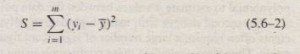This formula can be used to compute another measure of the quality of the curve fit, the coefficient of determination, also known as the r-squared value. It is defined as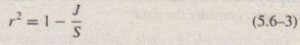For a perfect fit, J = 0 and thus r2 = I. Thus the closer r2 is to I, the better the fit. The largest r2 can be is I. The value of S indicates how much the data is spread around the mean, and the value of J indicates how much of the data spread is unaccounted for by the model. Thus the ratio J I5 indicates the fractional variation unaccounted for by the model. It is possible for J to be larger than 5, and thus it is possible for r2 to be negative. Such cases, however, are indicative of a very poor model that should not be used. As a rule of thumb, a good fit accounts for at least 99 percent of the data variation. This value corresponds to r2 ~ 0.99. For example, the following table gives the values of J, 5, and r2 for the first through
fourth-degree polynomials used to fit the data x = I, 2, 3, … , 9 and
y =5,6,10,20,28,33,34,36,42.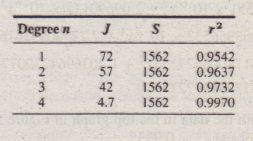Because the fourth-degree polynomial has the largest r2 value, it represents t data better than the representation from first- through third-degree poly nomia according to the ,.2 criterion. To calculate the values of Sand r2, add the following lines to the end of the script file shown bn pages 315 to 316.
mu = mean(y);
for k=1:4
S (k) = sum ( (y-mu) . “2) ;
r2 (k ) = 1 – J (k ) IS (k ) ;
end
S
r2

Regression and Numerical Accuracy
We mentioned that there are two dangers in using high-degree polynomials. The first danger is that high-degree polynomials often exhibit large excure ions between the data points, and thus we could get large errors if we were [0 use a high-degree polynomial to estimate y values between the data points. The second danger with using high-degree polynomials is that their coefficients often require a large number of significant figures to be represented accurately. Thus if you calculate these coefficients using the poly fit function and you intend to include these coefficients in a report, for example, then you should display them with the format long or format long e commands. As an example, consider the data:Using the format long command, a sixth-degree polynomial fit,
poly fit (x, y, 6), gives the result
y = -6.33551 X 10-9 x 6 + 2.09690135 x 10-6 x 5
– 1.9208956532 X 10-4 x 4 + 7.77770991616 X 10-3 x 3
– o.15 I780065271 53×2 + 1.20369642390774x
+0.0577394277217 (5.6-4)
It is plotted along with the data in the top graph in Figure 5.6-5. The J and r2 values are J = 0.1859 and r2 = 0.9935.

Figure 5.6-5 Effect of coefficient accuracy on a sixth-degree polynomial. The top graph shows the effect of 14 decimal-place accuracy. The bottom graph shows the effect of 8 decimal-place accuracy Now suppose we keep only the first eight decimal places of the coefficients. The resulting polynomial isThis polynomial is plotted in the bottom graph of Figure 5.6–5. Obviously, the polynomial deviates greatly from the data for the larger values of x. The fit is so poor as to be useless for values of x greater than 26 approximately. Polynomials whose coefficients have not been specified accurately enough are prone to large
errors at large values of x. High-degree polynomials have coefficients that not only must be displayed and stored with great accuracy but also are harder to compute accurately. As the polynomial degree increases, the number of linear equations to be solved also increases, and inaccuracies in the numerical solution of these equations become more significant. An alternative to using a high-degree polynomial is to fit two or more functions to the data. To illustrate this approach, we will fit two cubics because a cubic has greater flexibility than a quadratic but is less susceptible to numerical inaccuracies than higher-degree polynomials. Examining the data’ plot in

Figure 5.6-5, we see that the data has a bend near x = 5 and another bend near .r = 38. The transition between the convex and concave parts of the data appears to be around .r = 15. Thus we will fit one cubic over 0 S x < 15 and another over 15 < x S 40. The necessary script file follows.

xl [O:2:14);x2 = [16:2:40); .
y1 [0.1, 1.884, 2.732, 3.388, 3.346, 3, 2.644, 2.022j
y2 [1.65, 1.5838, 1.35, 1.0082, 0.718, 0.689, 0.4308,
0.203, 0.1652, -0.073, -0.002, -0.1122, 0.106);
x = [x1,x2);
y ,; [yl,y2);
% Create variables zl and z2 to generate the curve.
zl = [0:0.01:15); z2=[15:0.01:40);
% Fit two cubics.
wI = polyfit(x1,y1,3);
w2 = polyfit(x2,y2,3);
% Plot the results.
plot(zl,polyval(w1,zl),z2,polyval(w2,z2),x,y, ‘0’), …
xlabel (‘x’) ,ylabel (‘y’)
% Compute the coefficient of determination.
mu1 = mean (y l);
mu2 = mean (y2) ;
S = sum((y1-mul).”2) + sum((y2-mu2).”2)
J = sum((polyval(w1,x1)-y1) .”2) + sum( (polyval(w2,x2)-y2) .”2)
r2 = 1 – J/S

The values are S = 12.8618, J = 0.0868, and ,.2 = 0.9932, which indicates a very good fit. The plot is shown in Figure 5.6-6. The curves are not tangent, but they need not be for this example. However, some applicrions require the curves to be tangent, and in Section 7.4 we develop a method fur .uing rubies that are tangent. Here we estimated the transition point .r = 15 at which to separate the two cubics. You can experiment  with other values to improve the fit, although the fit we achieved is very good. If we want to estimate a value of y for 0 S .r S 15, we use the first cubic, which is found from w1 and isTo estimate a value of)’ for 15 < x S 40, we use the second cubic, which isfound from w2 and is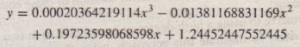Note that MATLAB reports the coefficients to 14 decimal places.
To demonstrate the robustness of the cubic polynomials, their coefficients were rounded off to eight decimal places. For these rounded coefficients

S = 12.8618, J = 0.0868, and r2 = 0.9932; these values are identical to the results obtained with the more accurate coefficients

Scaling the Data
The effect of computational errors in computing the coefficients can be lessened by properly scaling the x values. When the function poly f i t (x ,y r n) is executed, it will issue a warning message if the polynomial. degree n is greater than or equal to the number of data points (because there will not be enough equations for MATLAB to solve for the. coefficients), or if the vector x has repeated, or nearly repeated, points, or if the vector x needs centering and/or scaling. The alternate syntax [p, S , mu] = poly fit (x , y , n) finds the coefficients p of a polynomial of degree n in terms of the variable

x = (x – J-Lx)/ux

The output variable mu is a two-element vector, [/lx, uxJ, where /lx is the mean of x, and Ux is the standard deviation of x (the standard deviation is discussed in Chapter 7). You can scale be data yourself before using poly fit. Some common scaling methods areConstraining Models to Pass through a Given Point
Many applications require a model whose form is dictated by physical principles. For example, the force-extension model of a spring must pass through the origin (0, 0) because the spring exerts no force when it is unstretched. Thus a linear spring model f = a)x + a2 must have a zero value for a2. However, in general the poly fit function will give a nonzero value for a2 because of the scatter or measurement error that is usually present in the data. Linear Model. To obtain a zero-intercept model of the form y =a)x, we must derive the equation for a) from basic principles. The sum of the squared residuals in this case iswhich can be easily solved for al.
Quadratic Model For the quadratic rnodel, Y = a)x2 + a2X + a3, the coefficient a3 must be 0 for the curve to pass through the origin. The sum of the squared residuals in this case is In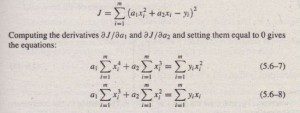These can be solved for al and a2. If the model is required to pass through a point not at the origin, say the point (xo, Yo), subtract Xo from all the x values, subtract Yo from all the Y values, and
then use the above equations to find the coefficients. The resulting equations will be of the formMultiple Linear Regression

Suppose that y is a linear function of two or more variables, XI, X2 For example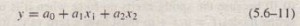To, find the coefficient values ao, a I, and a2 to fit a set of data (y, X” Xl) in the least squares sense, we can make use of the fact that the left-division method for solving linear equations uses the least squares method when the equation set is over determined (see Chapter 6, Section 6.5). To use this method, let /I be the number of data points and write the linear equation .in matrix form as’ follows.Linear-in-the-Parameters Regression
Sometimes we want to fit an expression that is neither a polynomial nor a function that can be converted to linear form by a logarithmic or other transformation. In some cases we can still do a least squares fit if the function is a linear expression in terms of its parameters. The following example illustrates the method.